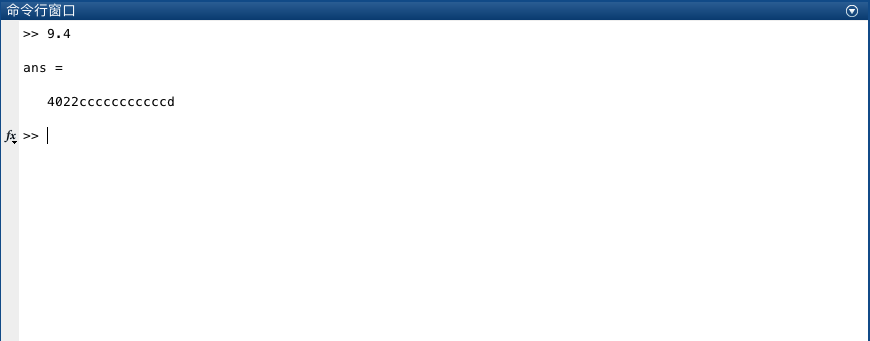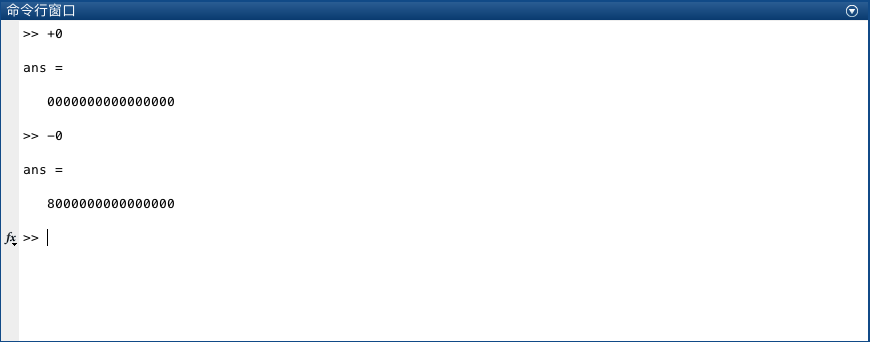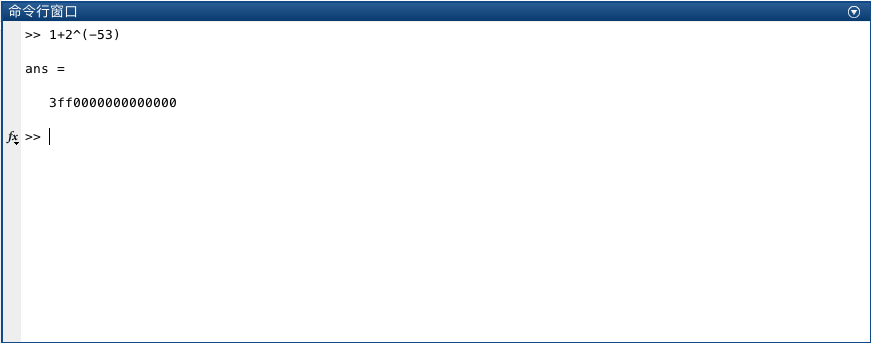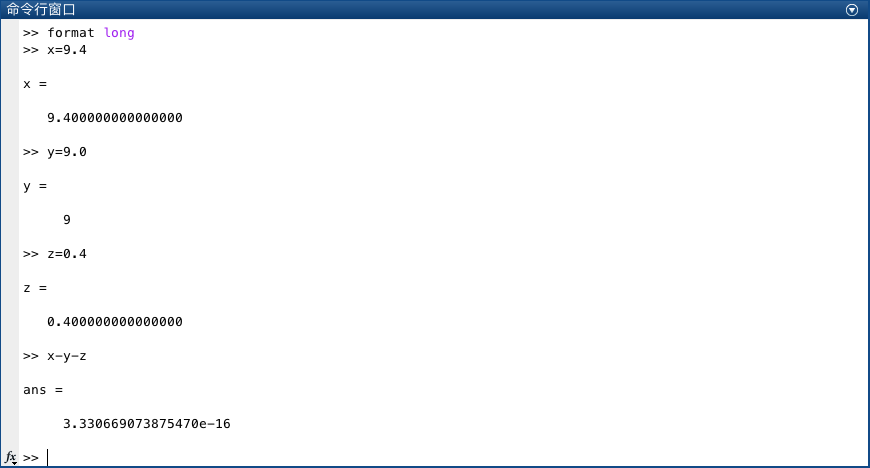Abstract: 本文介绍实数在计算机内的浮点数表示方法
Keywords: 浮点表示，IEEE754

# 实数的浮点表示

## 浮点格式

$$\pm 1.aaaaa\dots\times 10^m$$

$$\pm 1.aaaaa\dots\times 2^p$$

$$+1.01\times 2^{101}$$

0 00000101 01000000000000000000000

$$\varepsilon_{mach}=2^{-52}$$

• 截断，直接丢弃一定长度后面的数字而不管后面的数字是什么
• 舍入，十进制中四舍五入就是舍入

1. 53位是0的时候，舍去52后面的数字
2. 53位是1的时候，且52位之后不是 $1000\dots$ ，52位加1，然后舍去52位后面的数字
3. 当52位后面的数字是 $1000\dots$ 的时候，根据52位的数字选择是进位还是直接舍去，如果52位是1，则52位加1后截断否则直接截断

• 计算截断部分的十进制数
$$0.\overline{1100}\times2^3\times2^{-52}=0.4\times 2^{-48}$$
• 计算包含误差后的值
$$fl(9.4)=9.4+2^{-52}\times 2^{3}-0.4\times 2^{-48}\\ =9.4+0.2\times 2^{-49}$$

• 绝对误差： $|x-x_c|$
• 相对误差：$\frac{|x-x_c|}{x}$
其中 $x\neq 0$

$$\frac{|x-x_c|}{x}\leq \frac{1}{2}\varepsilon_{mach}$$

## 机器表示

$$se_1e_2\cdots e_11b_1b_2\cdots b_52$$

• 第一位s是符号位的个没什么说的
• 第二位开始的11位表示指数，为正数；考虑到指数也有正负，这里没有设置符号位，而是通过让指数去叠加 $2^{10}-1=1023$ 来得到，这样指数范围就是 $[-1022,1023]$ 之间。由于特殊目的，没有使用 $[0,2047]$ 这个我们后面会说
• 后面的 $b$ 就是尾数了| 0 | 01111111111 | 0000 $\cdots$ 0 |
|:——:|:——————:|:———————–:|
| 符号位 | 指数（加了1023） | 尾数52位 |

9.4的表示：9.4的二进制大家自己画画吧

+Inf 1/0 7FF0 0000 0000 0000
-Inf -1/0 FFF0 0000 0000 0000
Nan 0/0 FFFx xxxx xxxx xxxx

$$\pm0.b_1b_2\dots b_52\times 2^{-1022}$$## 浮点数加法

1. 将两个数字的对齐，所谓对齐就是对齐小数点。
2. 在特定的寄存器存储完成加法，寄存器能存储多于52的精度
3. 计算完成后进行舍入，变回52位尾数。

\begin{aligned} &1.\boxed{00\cdots 0} \times 2^0+1.\boxed{00\cdots 0}\times 2^{-53}\\ =&1.\boxed{0000000000000000000000000000000000000000000000000000}\times 2^0\\ +&0.\boxed{0000000000000000000000000000000000000000000000000000}1\times 2^0\\ &\hline\\ =&1.\boxed{0000000000000000000000000000000000000000000000000000}1\times 2^0\\ =&1.\boxed{0000000000000000000000000000000000000000000000000000}\times 2^0 \end{aligned}0%